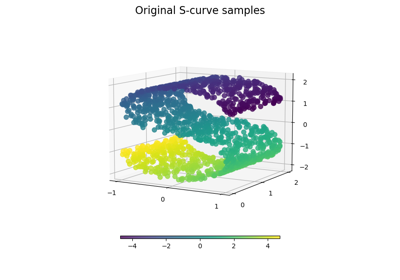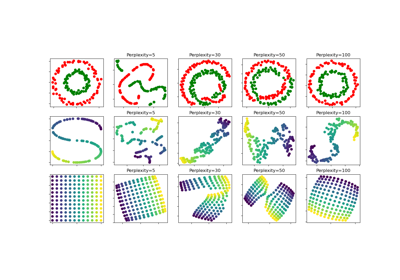# sklearn.datasets.make_s_curve¶

sklearn.datasets.make_s_curve(n_samples=100, *, noise=0.0, random_state=None)[source]

Generate an S curve dataset.

Read more in the User Guide.

Parameters:
n_samplesint, default=100

The number of sample points on the S curve.

noisefloat, default=0.0

The standard deviation of the gaussian noise.

random_stateint, RandomState instance or None, default=None

Determines random number generation for dataset creation. Pass an int for reproducible output across multiple function calls. See Glossary.

Returns:
Xndarray of shape (n_samples, 3)

The points.

tndarray of shape (n_samples,)

The univariate position of the sample according to the main dimension of the points in the manifold.

## Examples using sklearn.datasets.make_s_curve¶Comparison of Manifold Learning methods

Comparison of Manifold Learning methodst-SNE: The effect of various perplexity values on the shape

t-SNE: The effect of various perplexity values on the shape